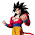## Shift signal in frequency domain¶

We need shift an signal for many cases, i.e. when we calculate cross correlation between signals, when we do beamforming to find out the direction of the energy comes, when we use back-projection to track the source of an earthquake, etc. One easy way to shift the signal is in the frequency domain. In this blog, I will show you how to shift an signal in the frequency domain. You can find the script at Qingkai's Github.

### The theory behind it¶

When we do a Fast Fourier Transform (FFT), we actually map a finite length of time domain samples into an equal length sequence of frequency domain samples.
$$X[k]=\sum_{n = 0}^{N−1} x[n]e^{\frac{−j2πnk}{N}}=A_ke^{jϕ_k}$$
A property of the Fourier transform is that, a delay in the time domain maps to a phase shift in the frequency domain. For the DFT, this property is:
$$x[n]↔X[k]$$$$x[n−D]↔e^{\frac{−j2πnkD}{N}}X[k]$$
This is to say, if we delay our input signal by D samples in the time domain, it is equavalent to each complex value in the FFT of the signal is multiplied by the constant $$e^{\frac{−j2πnkD}{N}}$$.
In :
import matplotlib.pyplot as plt
import numpy as np

In :
def nextpow2(i):
'''
Find the next power 2 number for FFT
'''

n = 1
while n < i: n *= 2
return n

def shift_signal_in_frequency_domain(datin, shift):
'''
This is function to shift a signal in frequency domain.
The idea is in the frequency domain,
we just multiply the signal with the phase shift.
'''
Nin = len(datin)

# get the next power 2 number for fft
N = nextpow2(Nin +np.max(np.abs(shift)))

# do the fft
fdatin = np.fft.fft(datin, N)

# get the phase shift for the signal, shift here is D in the above explaination
ik = np.array([2j*np.pi*k for k in xrange(0, N)]) / N
fshift = np.exp(-ik*shift)

# multiple the signal with the shift and transform it back to time domain
datout = np.real(np.fft.ifft(fshift * fdatin))

# only get the data have the same length as the input signal
datout = datout[0:Nin]

return datout


## Example¶

In :
Fs = 150.0;  # sampling rate
Ts = 1.0/Fs; # sampling interval
t = np.arange(0,1,Ts) # time vector

ff = 5;   # frequency of the signal

# let's generate a sine signal
y = np.sin(2*np.pi*ff*t)

# shift the signal in the frequency domain by 20 samples
y_shift = shift_signal_in_frequency_domain(y, -20)

plt.plot(y, label = 'y')
plt.plot(y_shift, label = 'y_shift')
plt.xlabel('Sample')
plt.ylabel('Amplitude')
plt.legend()
plt.show()


1.Hi Qingkai,

I never undertood why the frequency shift doesn't work for fractional shifts.
Would be willing to make a post on this?
Or give a reference?

Thanks
Erick Talarico

2.Well done! It is so well written and interactive. Keep writing such brilliant piece of work. Glad i came across this post. Last night even i saw similar wonderful Python tutorial on youtube so you can check that too for more detailed knowledge on Python.https://www.youtube.com/watch?v=HcsvDObzW2U